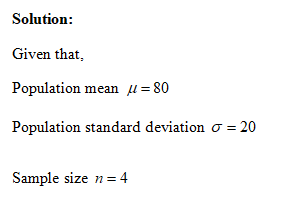# A random sample of n=4 scores is selected from a population with mu=80 and standard deviation=20. On average, how much difference would you expect between the sample mean and the population mean?

Question
21 views

A random sample of n=4 scores is selected from a population with mu=80 and standard deviation=20. On average, how much difference would you expect between the sample mean and the population mean?

check_circle

Step 1...

### Want to see the full answer?

See Solution

#### Want to see this answer and more?

Solutions are written by subject experts who are available 24/7. Questions are typically answered within 1 hour.*

See Solution
*Response times may vary by subject and question.
Tagged in